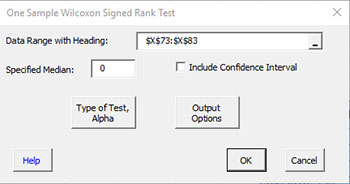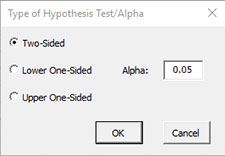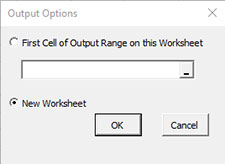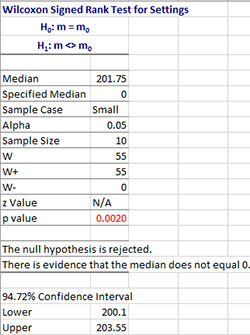# One Sample Wilcoxon Signed Rank Test HelpThis method is based on collecting a number of samples from a population with unknown median, m. The median is used in place of the average because the median is a better measure of the central tendency of the data than the average for non-normal data. The example below shows how to do this test using the SPC for Excel software.

Example (from Statistics and Data Analysis, by Ajit Tamhane and Dorothy Dunlop, Prentice-Hall, 2000)

A thermostat is used in an electrical device to check to the accuracy of the design setting of 200 °F. Ten thermostats were tested to determine their actual settings. The results are given below.

202.2, 203.4, 200.5, 202.5, 206.3, 198.0, 203.7, 200.8, 201.3, 199.0

We want to use these results to see if the median setting is significantly different from the design setting of 200.

1. Enter the results into an Excel worksheet as shown below. The data can be downloaded at this link.2. Select the data and heading.

3. Select “NonParametric” from the “Statistical Tools” panel on the SPC for Excel ribbon.

4. Select the “One Sample Wilcoxon Signed Rank Test” option and then OK.• Data Range with Heading: enter the range containing the data with headings default is the range selected on the worksheet.
• Specified Median: enter the specified median; 0 is the default.
• Include Confidence Interval: check if you want the confidence interval displayed; default is not to display the confidence interval.
• Type of Test, Alpha: the input form below is displayed if this option is selected.• Select the Type of Test: two-sided; lower one-sided, or upper one-sided, the default is two-sided.
• Alpha: this is the confidence level; 1-alpha is the confidence interval, default is 0.05 or 95% confidence interval.
• Output Options: the input form below is displayed if this option is selected.• First Cell of Output Range on this Worksheet: Select this option if you want the output on an existing worksheet; then select the first cell of the range where you want the output placed; a check is made to ensure that no existing data are overwritten in the worksheet.
• New Worksheet: Select this option if you want the results on a new worksheet.
• Select OK to generate the results.
• Select Cancel to end the program.

One Sample Wilcoxon Signed Rank Test Output

The output from the One Sample Wilcoxon Signed Rank test is given below.• The null hypothesis (m is median) and the alternate hypothesis (two sided in this example) are listed at the top.
• Median: Calculated median from the data.
• Specified Median: Specified median entered by user.
• Alpha: Value of alpha entered by user.
• Sample Case: Calculations; if sample size < 20, the null distribution of the Wilcoxon Signed Rank Statistic is used (small case); if sample size ≥ 20, the normal distribution is used (large case).
• Sample Size : Sample size not including values equal to specified median.
• W: W+ – W-.
• W+: Sum of ranks of positive values.
• W-: Sum of ranks of negative values.
• z value: Calculated z value for large sample case; N/A for small sample case.
• p value: Calculated p value (will be red if ≤alpha).

The conclusions, based on value of p and alpha, are given. Reject the null hypothesis if p value < alpha.

Notes:

• Any values equal to the median are not included; this reduces the effective sample size but should not be an issue as long as there are not too many values equal to the median.
• The software uses the null distribution of the Wilcoxon rank signed statistic when the sample size is less than 20 and uses a normal approximation when the sample size is greater than or equal to 20.
• Exact confidence intervals cannot usually be calculated. The confidence interval given is two-sided and based on a normal distribution approximation with a continuity correction.
• The calculated median is the median of the Walsh averages.